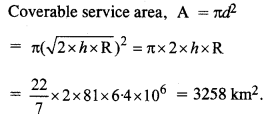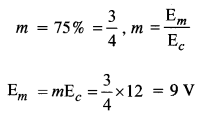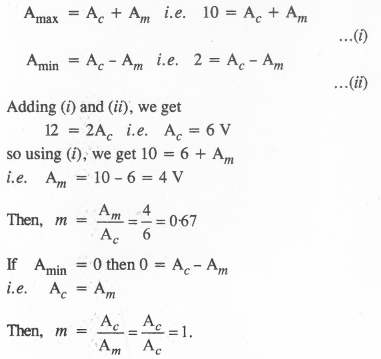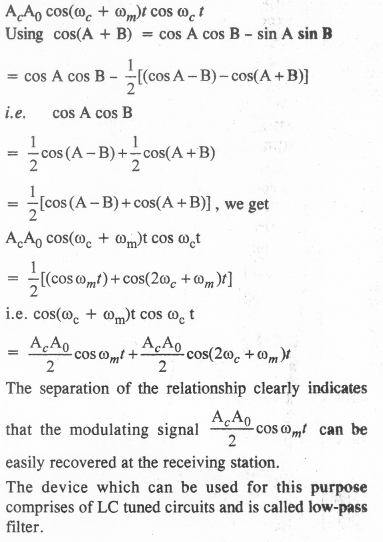# NCERT Solutions Class 12 Physics Chapter-15 (Communication Systems)

NCERT Solutions Class 12 Physics from class 12th Students will get the answers of Chapter-15 (Communication Systems) This chapter will help you to learn the basics and you should expect at least one question in your exam from this chapter.
We have given the answers of all the questions of NCERT Board Physics Textbook in very easy language, which will be very easy for the students to understand and remember so that you can pass with good marks in your examination.

Class 12 Physics

Chapter-15 (Communication Systems)

Questions and answers given in practice

Chapter-15 (Communication Systems)

Question 1.

At which of the following frequency/frequencies the communication will not be reliable for a receiver situated beyond the horizon:
(a) 10 kHz
(b) 10 MHz
(c) 1 GHz
(d) 1000 GHz

(b) is correct. Here (c) and (d) frequencies have high penetration power so the earth will absorb them. Radiation (a) of 10 kHz will suffer from the problem of the size of the antenna.

Question 2.
Frequencies in the UHF range normally propagate by means of
(a) ground waves
(b) sky waves
(c) surface waves
(d) space waves.

(d) space waves.

Question 3.
Digital signals (i) do not provide a continuous set of values, (ii) represent values as discrete steps, (Hi) can utilize the only binary system, and (iv) can utilize decimal as well as a binary system. Which of the following options is true :
(a) Only (i) and (ii).
(b) Only (ii) and (iii).
(c) Only (i), (ii) and (iii), but not (iv).
(d) AH the above (i) to (iv).

(c) is correct because the decimal system is concerned with continuous values (i) to (iii).

Question 4.
Is it necessary for a transmitting antenna to be at the same height as that of the receiving antenna for line-of-sight communication? A TV transmitting antenna is 81 m tall. How much service area can it cover if the receiving antenna is at the ground level?

For line-of-sight communication, it is necessary that the transmitting antenna and receiving antenna should be eye to eye but it is not necessary that they should be at the same height.Question 5.
A carrier wave of peak voltage 12 V is used to transmit a message signal. What should be the peak voltage of the modulating signal in order to have a modulation index of 75%?Question 7.
For an amplitude modulated wave, the maximum amplitude is found to be 10 V while the minimum amplitude is found to be 2 V. Determine the modulation index μ. What would be the value of μ if the minimum amplitude is zero V?